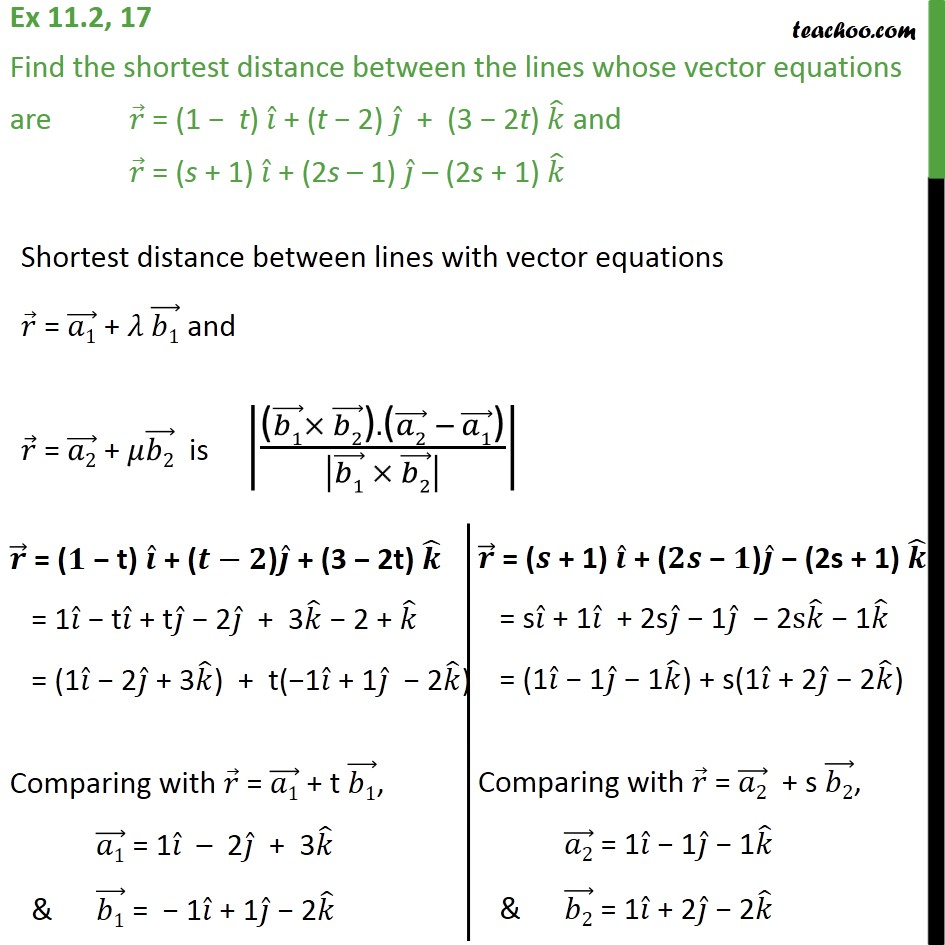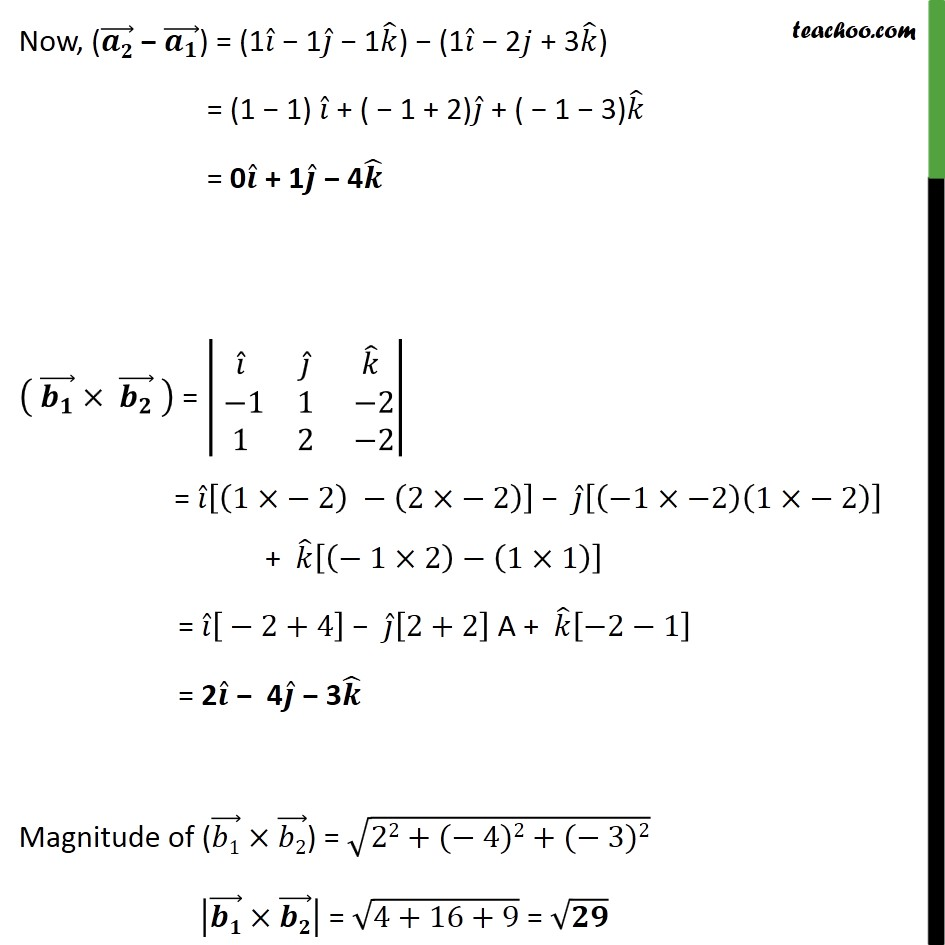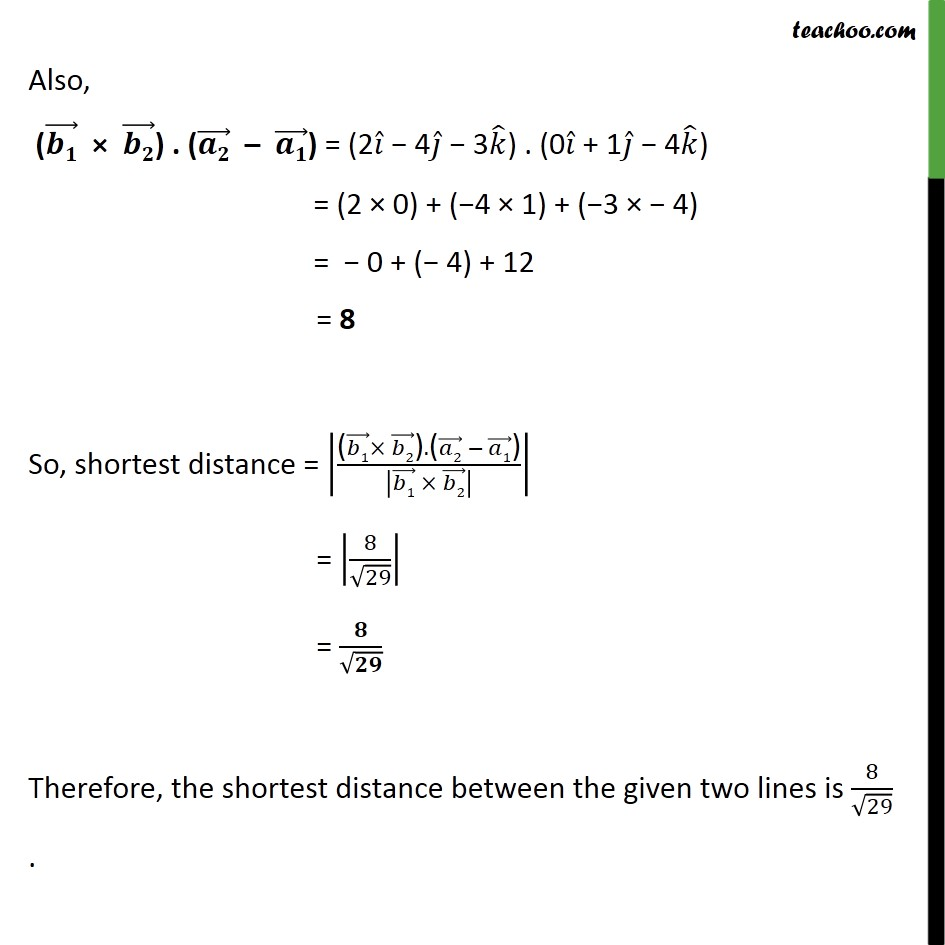1. Chapter 11 Class 12 Three Dimensional Geometry
2. Serial order wise
3. Ex 11.2

Transcript

Ex 11.2, 17 Find the shortest distance between the lines whose vector equations are 𝑟﷯ = (1 − t) 𝑖﷯ + (t − 2) 𝑗﷯ + (3 − 2t) 𝑘﷯ and 𝑟﷯ = (s + 1) 𝑖﷯ + (2s – 1) 𝑗﷯ – (2s + 1) 𝑘﷯ Shortest distance between lines with vector equations 𝑟﷯ = 𝑎1﷯ + 𝜆 𝑏1﷯ and 𝑟﷯ = 𝑎2﷯ + 𝜇 𝑏2﷯ is ( 𝑏1﷯× 𝑏2﷯).( 𝑎2﷯ − 𝑎1﷯)﷮ 𝑏1﷯ × 𝑏2﷯﷯﷯﷯ Now, ( 𝒂𝟐﷯ − 𝒂﷮𝟏﷯﷯) = (1 𝑖﷯ − 1 𝑗﷯ − 1 𝑘﷯) − (1 𝑖﷯ − 2𝑗 + 3 𝑘﷯) = (1 − 1) 𝑖﷯ + ( − 1 + 2) 𝑗﷯ + ( − 1 − 3) 𝑘﷯ = 0 𝒊﷯ + 1 𝒋﷯ − 4 𝒌﷯ 𝒃﷮𝟏﷯﷯× 𝒃﷮𝟐﷯﷯ ﷯ = 𝑖﷯﷮ 𝑗﷯﷮ 𝑘﷯﷮ −1﷮1﷮ −2﷮1﷮2﷮ −2﷯﷯ = 𝑖﷯ 1×− 2﷯ − 2×− 2﷯﷯ − 𝑗﷯ −1×−2﷯ 1×− 2﷯﷯ + 𝑘﷯ − 1×2﷯− 1×1﷯﷯ = 𝑖﷯ − 2+4﷯ − 𝑗﷯ 2+2﷯ A + 𝑘﷯ −2−1﷯ = 2 𝒊﷯ − 4 𝒋﷯ − 3 𝒌﷯ Magnitude of ( 𝑏1﷯× 𝑏2﷯) = ﷮22+(− 4)2+(− 3)2﷯ 𝒃𝟏﷯× 𝒃𝟐﷯﷯ = ﷮4+16+9﷯ = ﷮𝟐𝟗﷯

Ex 11.2